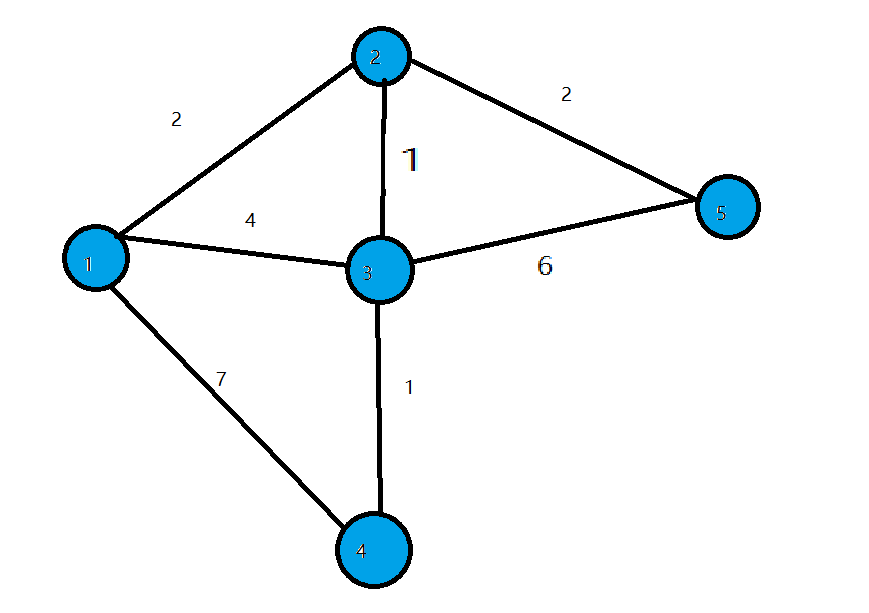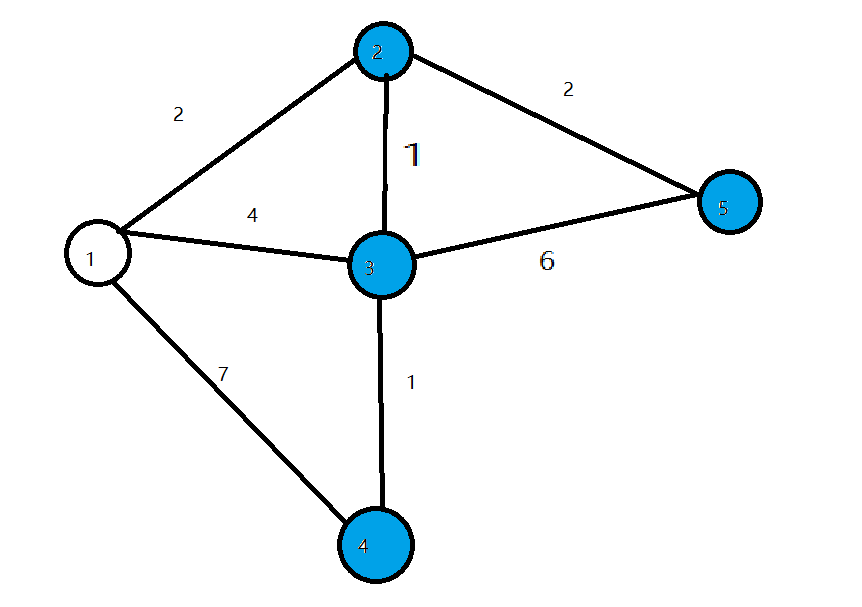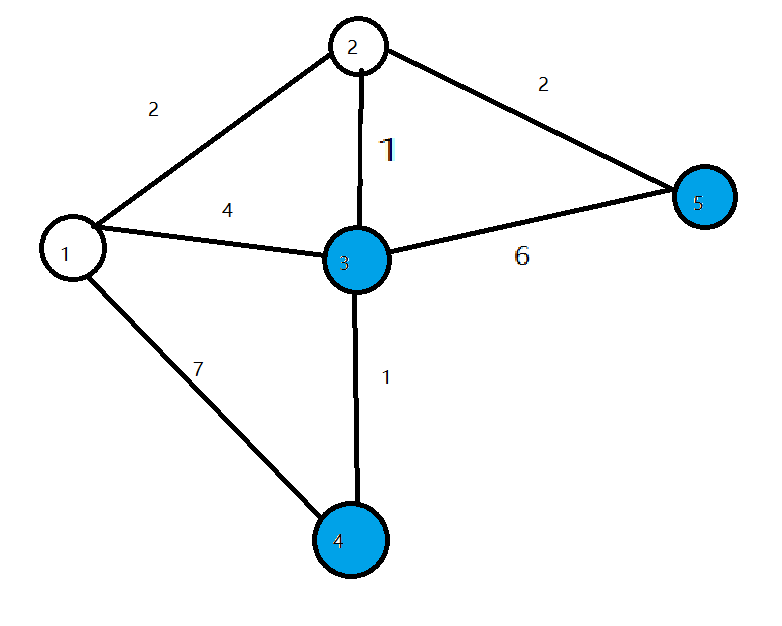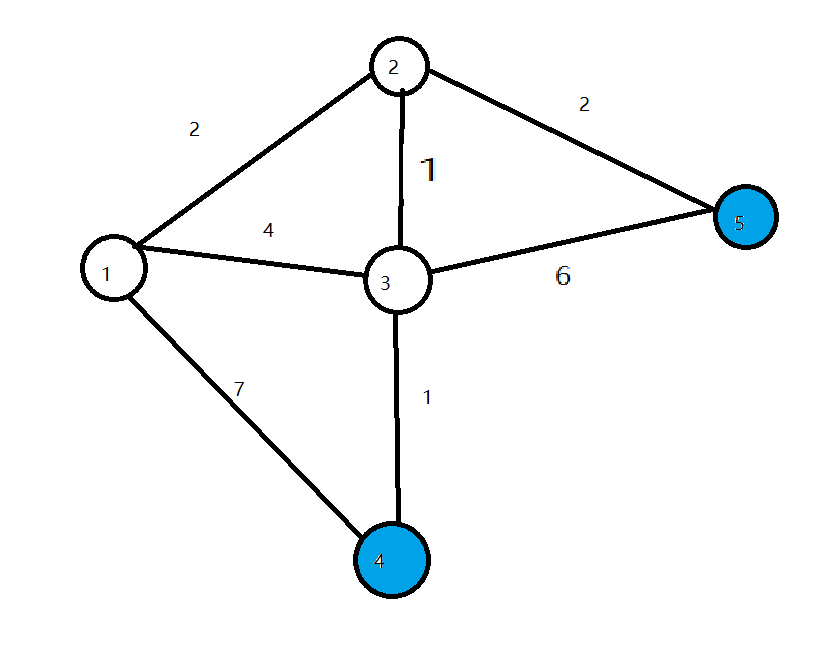# 「算法笔记」Dijkstra

### 前言

• $SPFA​$算法由于它上限 $O(NM) = O(VE)​$的时间复杂度,被卡掉的几率很大.在算法竞赛中,我们需要一个更稳定的算法:$dijkstra​$.

### 什么是$dijkstra$?

• $dijkstra​$是一种单源最短路径算法,时间复杂度上限为$O(n^2)​$(朴素),在实际应用中较为稳定$;​$加上堆优化之后更是具有$O((n+m)\log_{2}n)​$的时间复杂度,在稠密图中有不俗的表现.

### $dijkstra$的原理/流程?

• $dijkstra$本质上的思想是贪心,它只适用于不含负权边的图.
• 我们把点分成两类,一类是已经确定最短路径的点,称为”白点”,另一类是未确定最短路径的点,称为”蓝点”
• $dijkstra$的流程如下$:$
• $1.$ 初始化$dis[start] = 0,$其余节点的$dis$值为无穷大.
• $2.$ 找一个$dis$值最小的蓝点$x,$把节点$x$变成白点.
• $3.$ 遍历$x$的所有出边$(x,y,z),$若$dis[y] > dis[x] + z,$则令$dis[y] = dis[x] + z$
• $4.$ 重复$2,3$两步,直到所有点都成为白点$.$
• 时间复杂度为$O(n^2)$

### $dijkstra$为什么是正确的

• 当所有边长都是非负数的时候,全局最小值不可能再被其他节点更新.所以在第$2$步中找出的蓝点$x$必然满足$:dis[x]$已经是起点到$x$的最短路径$.$我们不断选择全局最小值进行标记和拓展,最终可以得到起点到每个节点的最短路径的长度

### 图解

• (令$start = 1$)
• 开始时我们把$dis[start]$初始化为$0$,其余点初始化为$inf$• 第一轮循环找到$dis$值最小的点$1$,将$1$变成白点,对所有与$1$相连的蓝点的$dis$值进行修改,使得$dis=2,dis=4,dis=7$• 第二轮循环找到$dis$值最小的点$2$,将$2$变成白点,对所有与$2$相连的蓝点的$dis$值进行修改,使得$dis=3,dis=4$• 第三轮循环找到$dis$值最小的点$3$,将$3$变成白点,对所有与$2$相连的蓝点的$dis$值进行修改,使得$dis=4$• 接下来两轮循环分别将$4,5$设为白点,算法结束,求出所有点的最短路径
• 时间复杂度$O(n^2)$

### 为什么$dijkstra$不能处理有负权边的情况?

• 我们来看下面这张图• $2$到$3$的边权为$-4$,显然从$1$到$3$的最短路径为$-2$ $(1->2->3).$但在循环开始时程序会找到当前$dis$值最小的点$3$,并标记它为白点.
• 这时的$dis=1,$然而$1$并不是起点到$3$的最短路径.因为$3$已经被标为白点,所以$dis$不会再被修改了.我们在边权存在负数的情况下得到了错误的答案.

### $dijkstra$的堆优化?

• 观察$dijkstra$的流程,发现步骤$2$可以优化
• 怎么优化呢?
• 我会zkw线段树!我会斐波那契堆!
• 我会堆!
• 我们可以用堆对$dis$数组进行维护,用$O(\log_{2}n)$的时间取出堆顶元素并删除,用$O(\log_{2}n)$遍历每条边,总复杂度$O((n+m)\log_{2}n)$

• 范例代码:

### 例题

• 入门模板:P3371
• 进阶模板:P4779
• 其余例题请右转洛谷 题库,搜索”最短路”

### 后记

• 本文部分内容摘自李煜东《算法竞赛进阶指南》和《信息学竞赛一本通》
• 友情提示:正权图请使用$dijkstra$算法,负权图请使用$SPFA$算法
• 感谢洛谷 各位管理员提供的平台